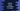# Python program to merge a dictionary to a tuple## Python program to merge a dictionary to a tuple:

We know that Python has four different collection data types: list, dictionary, tuple and sets. Dictionary and tuple, both are different collection data types. So, adding a dictionary to the end of a tuple is not straightforward.

In this post, we will learn how to merge a dictionary or append a dictionary to a tuple in different ways.

### Method 1: By converting the tuple to a list:

Tuple is immutable and it is an ordered collection of items. As it is immutable, we can’t modify a tuple. But, we can convert a tuple to a list and vice versa.

So, we can simply convert a tuple to a list, add the dictionary to the end of the list and convert the list back to a tuple.

• Convert the tuple to a list
• Add the dictionary to the end of the list.
• Convert the list back to a tuple.

Below is the complete program,

``````given_tuple = (1, 2, 3, 4, 5)
given_dic = {'one': 1, 'two': 2}

print(f'Given tuple: {given_tuple}')
print(f'Given dictionary: {given_dic}')

converted_list = list(given_tuple)
converted_list.append(given_dic)
given_tuple = tuple(converted_list)

print(f'Final tuple: {given_tuple}')``````

Here,

• given_tuple is the given tuple. given_dic is the dictionary.
• It is printing the tuple and dictionary at the start of the program.
• The tuple given_tuple is converted to a list and assigned to the list converted_list by using list().
• Then we are appending the dictionary to the end of the list converted_list by using the append() method.
• Finally, the list is converted back to a tuple by using the tuple() method. This value is assigned to given_tuple.
• The last line is printing the tuple.
``````Given tuple: (1, 2, 3, 4, 5)
Given dictionary: {'one': 1, 'two': 2}
Final tuple: (1, 2, 3, 4, 5, {'one': 1, 'two': 2})``````

### Method 2: By using the + operator:

We can also use the addition operator + to append a dictionary to the end of a tuple. It creates a new tuple and returns that tuple. We can assign this to the original tuple variable. But, we have to insert the dictionary as an element of another tuple. Otherwise, we can’t add it to the end.

Below is the complete program:

``````given_tuple = (1, 2, 3, 4, 5)
given_dic = {'one': 1, 'two': 2}

print(f'Given tuple: {given_tuple}')
print(f'Given dictionary: {given_dic}')

given_tuple = given_tuple + (given_dic, )

print(f'Final tuple: {given_tuple}')``````

It will print the below output:

``````Given tuple: (1, 2, 3, 4, 5)
Given dictionary: {'one': 1, 'two': 2}
Final tuple: (1, 2, 3, 4, 5, {'one': 1, 'two': 2})``````

### Conclusion:

You can use any of these two methods to append a dictionary to the end of a tuple. The first method takes three steps and the second method takes only one step. Both of these methods create a different tuple as tuple is immutable in Python.